## INTRODUCTION

Measurement of TCO2 is useful in determining the acid—base status of a patient. The TCO2 in serum or plasma exists in three major chemical forms: 95% as bicarbonate anion, 3% as dissolved CO2, and 2% as carbamino derivatives of plasma protein.1 Other quantitatively minor forms are carbonic acid and carbonate ions. The use of the term “bicarbonate” to describe the measured parameter is not correct since the measured TCO2 includes dissolved CO2, carbamino compounds, and carbonic acid and not strictly bicarbonate. Some laboratories use the term “TCO2” to describe the measured bicarbonate. Two commonly employed methods to quantitate TCO2 content in serum or plasma involve acidification of the sample to convert all carbon dioxide forms to CO2 gas and measure the amount of gas formed using PCO2 electrode. The second method utilizes the enzymatic reaction of bicarbonate with phosphoenolpyruvate carboxylase coupled to a spectrophotometric indicator reaction. Most assays that specifically measure bicarbonate have reagents at an alkaline pH. This converts other forms of CO2 to bicarbonate, so these assays are also measuring total CO2. Bicarbonate can also be calculated from blood—gas analysis using the Henderson—Hasselbalch equation. The equation used to calculate bicarbonate is [HCO3] = αPCO2 (antilog [pH − pKa]). This equation is based on the assumption that the dissociation constant (pKa) and solubility coefficient (α) are 6.1 and 0.03 respectively. However, pKa is affected by changes in pH, ionic strength, and temperature while the values of solubility coefficient (α) vary with the composition of the solution, such as the presence of increased salts proteins or lipids.2 Therefore, the calculated bicarbonate values may have significant error under certain circumstances irrespective of the sample type, making the reliability questionable. Previously done studies to assess the agreement between calculated bicarbonate and measured TCO2 have shown good agreement in some studies3-5 while revealing conflicting results in others.6-8 The objective of this study is to compare and assess the degree of agreement between the measured TCO2 and calculated bicarbonate and also whether they can be used interchangeably.

## MATERIALS AND METHODS

We prospectively analyzed 89 ABG samples requested for calculated bicarbonate and measured TCO2 from venous blood samples drawn simultaneously from the same participants between November 2016—April 2017. Out of 89 participants, 54 were males within the age range (9—68 years) and 35 were females within the age range (11—66 years). Measured TCO2 results ranged from 5.7—39.9 mmol/L (mean 23.45 mmol/L), while calculated bicarbonate ranged from 9—40 mmol/L (mean 24.36 mmol/L).

The TCO2 was analyzed in venous sample by the Vitros ECO2 Slide method in Vitros 4600 Chemistry System analyzer.9 The bicarbonate reagent slide utilizes the enzymatic method whereby the high pH in the spreading layer ensures that essentially all CO2 in the sample is in the bicarbonate form. The bicarbonate then diffuses to the gel layer and is used to carboxylate phosphoenolpyruvate in the presence of phosphoenolpyruvate carboxylase to form oxaloacetate and inorganic phosphate. The final reaction involves the malate dehydrogenase-catalyzed oxidation of nicotinamide adenine dinucleotide (NADH) and reduction of oxaloacetate to produce NAD+ and malate. The slide is incubated at 37°C. The concentration of CO2 in the sample is determined by measuring the absorbance of the unreacted NADH by reflectance spectrophotometry.

Bicarbonate was calculated by the Roche Cobas b 221 Blood—Gas Analyzer using the Henderson—Hasselbalch equation. The equation used to calculate bicarbonate is as follows [HCO3] = αPCO2 (antilog [pH − pKa]). This equation is based on the assumption that pKa is the dissociation constant for carbonic acid (equal to 6.1 for blood at 37°C) and α is the solubility coefficient for CO2 gas (equal to 0.03 for plasma at 37°C).

### Statistical Analysis

The statistical analysis was performed using Microsoft Excel 2007.

(a) Paired t-test was used to compare all participants included in the study between the calculated bicarbonate from ABG and measured TCO2. The paired t-test was used since all parameters used in the study are numerical, all participants came from same population, and all had paired results.

(b) The comparison plot/least square linear regression analysis was used for comparison of calculated bicarbonate and measured TCO2. The values obtained by the measured TCO2 are plotted on the y-axis and the calculated bicarbonate from ABG is plotted on the x-axis. A line of “best fit” is drawn in such a way that there are minimum squares of distance of all the data points from the line chosen. This line is called “least squares line”. This graph/line has the following equation: y = a + bx; where “y” is test method, where “a” is called y intercept (the point/value at which the line cuts the y axis or y value).

(c) The Bland—Altman plot/difference plot was used for comparison of calculated bicarbonate and measured TCO2. This was analyzed with the averages of measured TCO2 and calculated bicarbonate on the x-axis and the differences between the two in mmol/L, plotted on the y-axis.

## RESULTS

Table 1 shows the comparison of all participants included in the study by Paired t-test, between the calculated bicarbonate from ABG and measured TCO2. A statistically significant difference (p< 0.05) was observed between calculated bicarbonate from ABG and measured TCO2 when all participants included in the study independent of age, sex, and race were compared.

##### Table 1

Comparison of all participants included in the study by paired t-test between the calculated bicarbonate and measured TCO2

Calculated bicarbonate from ABG (arterial blood)Measured TCO2—venous bloodp-value
Total no of participants8989<0.001
Mean23.4524.36
SD7.016.36
SE2.482.58
SD: Standard deviation; SE: Standard error

Graph 1 shows the comparison of calculated bicarbonate and measured TCO2 by comparison plot/least square linear regression analysis. The values obtained by the measured TCO2 are plotted on the y-axis and the calculated bicarbonate from ABG is plotted on the x-axis. Measured TCO2 results ranged from 5.7—39.9 mmol/L (mean 23.45 mmol/L), while calculated bicarbonate ranged from 9—40 mmol/L (mean 24.36 mmol/L). The values of TCO2 and bicarbonate correlated well (r = 0.95, p < 0.001), with the correlation given by the equation, y = 0.884x + 3.605.

##### Graph 1

Comparison of calculated bicarbonate and measured TCO2 by comparison plot/least square linear regression analysis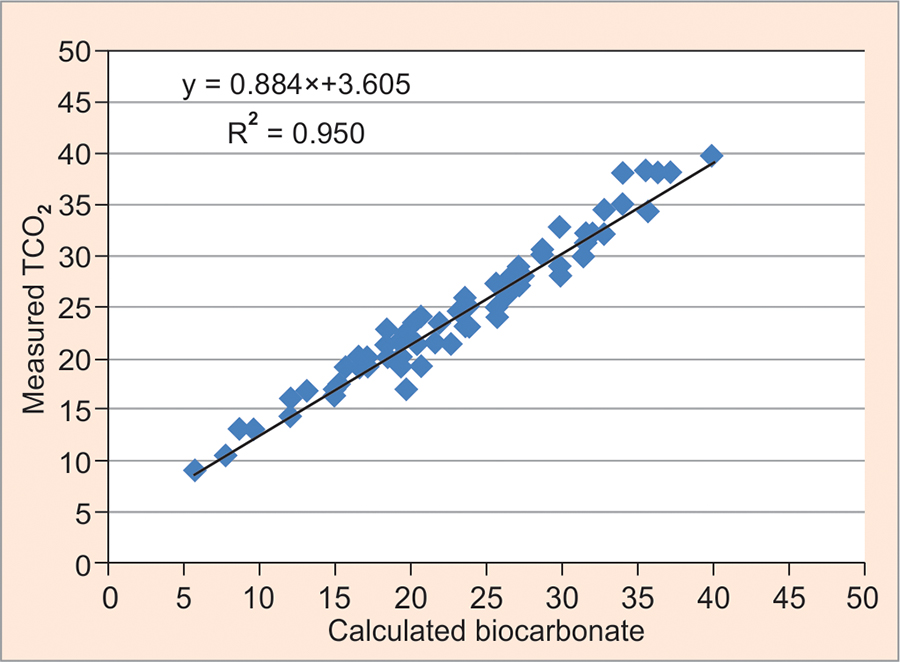Graph 2 shows the Bland—Altman plot/difference plot. This was analyzed with the averages of measured TCO2 and calculated bicarbonate on the x-axis and the differences between the two in mmol/L, plotted on the y-axis. The upper and lower LOA were calculated from bias ± 1.96 SD. The span was calculated from the high limit and low limit values. The bias obtained was —0.9 mmol/L and the SD was 1.62 mmol/L. The LOA were —4.1 to 2.3 mmol/L, with a span of 6.4 mmol/L. Out of the 89 values, 85 (95.05%) were within the LOA.

##### Graph 2

The Bland—Altman plot/difference plot analyzed with the averages of measured TCO2 and calculated bicarbonate on the x-axis and the differences between the two in mmol/L are plotted on the y-axis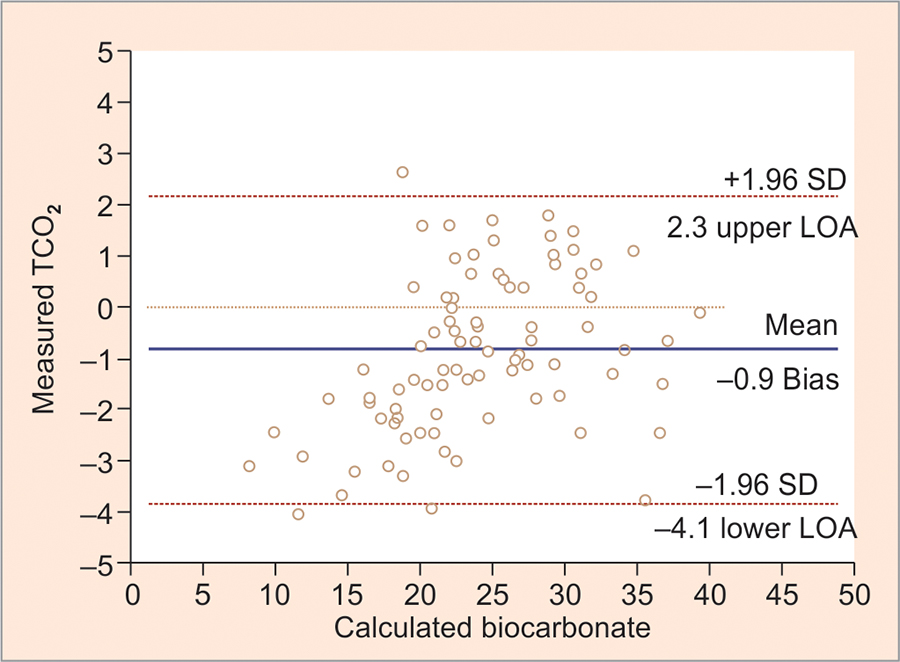Table 2 shows the comparison of participants divided into 3 subgroups based on the average value of bicarbonate [<20 mmol/L (group I), 20—26 mmol/L (group II) and >26 mmol/L (group III)] included in the study by paired t-test between the calculated bicarbonate and measured TCO2. A statistically significant difference (p < 0.001) was observed between calculated bicarbonate and measured TCO2 in the groups I and II, but a statistically insignificant p-value (p-value > 0.05) was observed in the group III.

##### Table 2

Comparison of participants divided into three subgroups based on the average value of bicarbonate <20 mmol/L (group I), 20—26 mmol/L (group II), and >26 mmol/L (group III) included in the study by paired t-test between the calculated bicarbonate and measured TCO2

Average bicarbonate (mmol/L)No. of participantsMean of calculated bicarbonateMean of measured TCO2Average meanp-value
<202415.1517.1516.15<0.001
20–263322.3422.9822.660.01
>263230.8331.1831.010.16
##### Graph 3

The Bland—Altman plot analysis with the averages of measured TCO2 and calculated bicarbonate (<20 mmol/L) on the x-axis and the differences between the two in mmol/L are plotted on the y-axis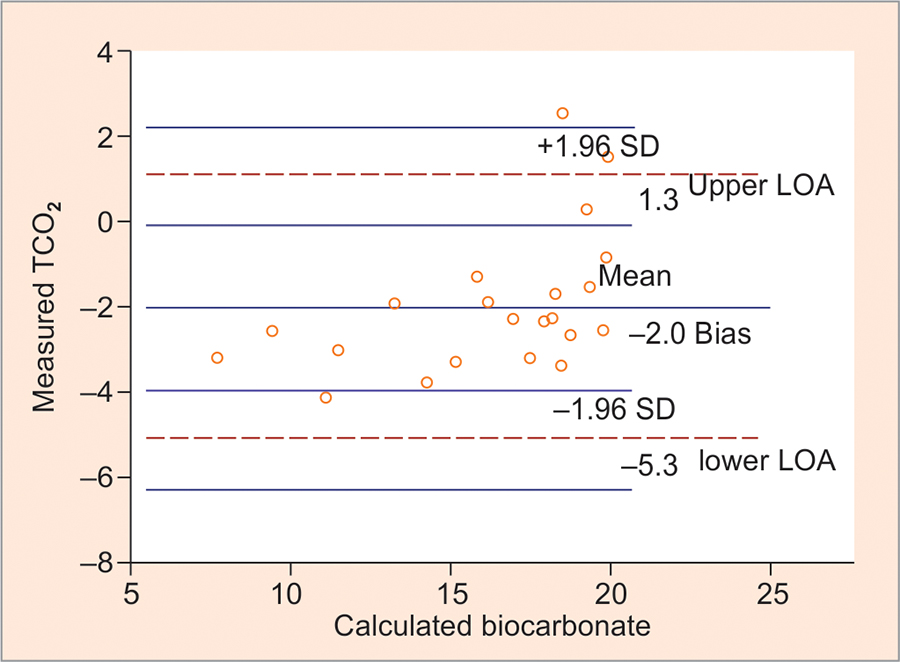##### Graph 4

The Bland—Altman plot analysis with the averages of measured TCO2 and calculated bicarbonate (20—26 mmol/L) on the x-axis and the differences between the two in mmol/L are plotted on the y-axis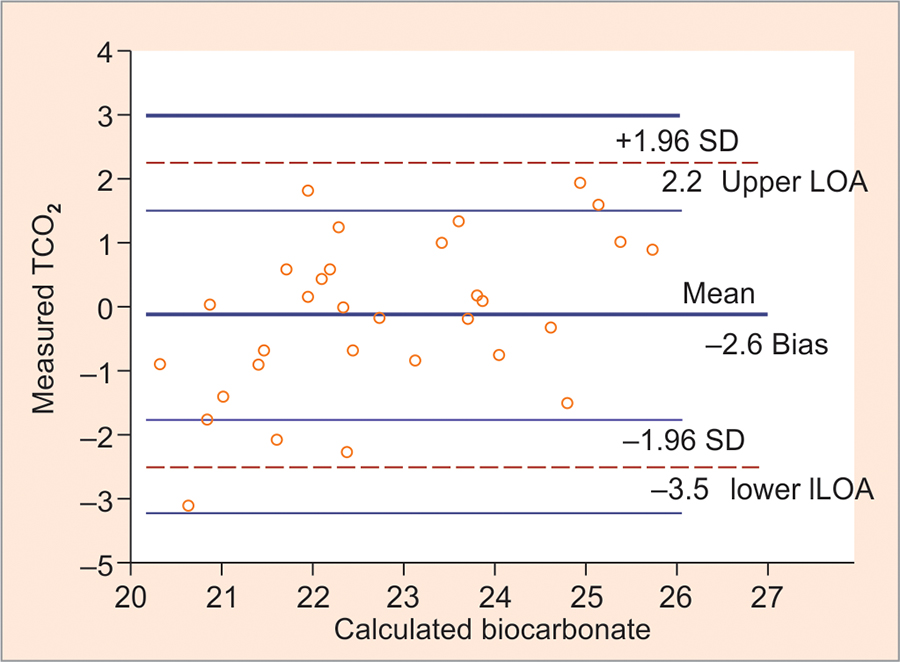Graphs 3 to 5 show the Bland—Altman plot analysis with the average value of measured TCO2 and calculated bicarbonate [< 20 mmol/L (group I), 20—26 mmol/L (group II), and 26—40 mmol/L(group III)] on the x-axis and the differences between the two in mmol/L plotted on the y-axis respectively.

##### Graph 5

The Bland—Altman plot analysis with the averages of measured TCO2 and calculated bicarbonate (>26 mmol/L) on the x-axis and the differences between the two in mmol/L are plotted on the y-axis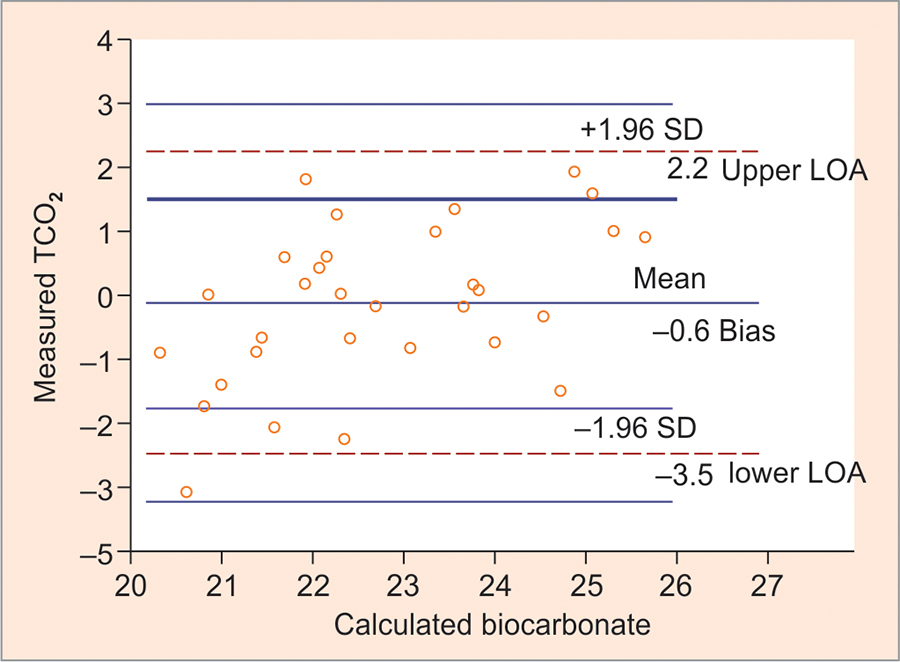## DISCUSSION

The agreement between calculated bicarbonate from venous sample and measured TCO2 from ABG have shown good agreement in some studies3-5 while revealing conflicting results in others.6-8 The objective is to find out whether the values obtained by both the methods can be used interchangeably in our laboratory.

Theoretically measured TCO2 is slightly higher than calculated bicarbonate. This is because the measured TCO2 includes an additional nonbicarbonate CO2 component which amounts to around 1.2 mmol/L. The other reason is that the serum or plasma sample used to measure TCO2 is derived from venous blood, which in itself has a slightly higher bicarbonate concentration than that of the arterial blood. These theoretical reasons in themselves amount to approximately 2.0 mmol/L difference between calculated and measured bicarbonate.10-12

The first step in assessing agreement between measured and calculated bicarbonate by correlation coefficient showed a good correlation with r = 0.950 with p < 0.05. The correlation coefficient to assess the agreement depends not only on the correlation coefficient, but also on the range of values, so a wide range of values will show a good correlation even if the values are in poor agreement.

By Bland—Altman analysis, the bias and SD obtained were —0.9 and 1.62 mmol/L respectively. The LOA were —4.1 to 2.3 mmol/L, with a span of 6.4 mmol/L. Out of the 89 values, 85 (95.05%) were within the LOA. Then Story and Poustie's criteria were used to assess the degree of agreement between calculated bicarbonate and measured TCO2.13 The criteria proposed for agreement are: (i) The bias or difference between calculated TCO2 and measured bicarbonate should be less than ±1 mmol/L, (ii) the LOA between the methods should range within a bias ±2 mmol/L or within a total span of 4 mmol/L, to be clinically insignificant. The results of our study showed a bias of —0.9 mmol/L, which fulfilled the first criteria, but the LOA were —4.1 to 2.3 mmol/L, with a span of 6.4 mmol/L, which did not fulfill the second criteria. Despite good statistical correlation obtained by paired t-test and linear regression analysis, the application of criteria proposed by Story and Poustie for agreement between TCO2 and HCO3 is not fully satisfied.

The participants were subgrouped into three groups based on the average value of measured TCO2 and calculated bicarbonate as follows, average bicarbonate <20 mmol/L (group I), 20—26 mmol/L (group II) and 26—40 mmol/L (group III) independent of age and sex. On application of the criteria of Story and Poustie, the first subgroup did not fulfill both the criteria but the 2nd and 3rd subgroups fulfilled the first criteria, but not the second criteria. Hence, the poor agreement between the two methods was observed at lower bicarbonate concentration, especially at bicarbonate concentration at <20 mmol/L.

The discrepancy in values between measured TCO2 and calculated bicarbonate can be explained because of usage of a constant pKa of 6.1 in the Henderson—Hasselbalch equation, i.e., used to calculate the bicarbonate. The study of Flear et al14 showed that pKa values varied considerably by more than 0.06 in healthy volunteers and in very ill patients and hence, an error of approximately ± 60% was noted in calculated bicarbonate based on constant pKa of 6.1. Another study by O'Leary and Langton found a significant decrease of the pKa in patients considered to have metabolic acidosis when compared with patients with bicarbonate concentration within the reference interval.6 The findings in our study also noted that majority of values falling outside the LOA occurred at bicarbonate concentration <20 mmol/L, which seem to be in agreement with O'Leary and Langton.

The other cause of discrepancy in results could be due to preanalytical factors, such as sample collection and handling like inadequate mixing, acidification, nonmaintenance of anaerobic condition, and dilution of ABG samples with excess heparin that can decrease the pH and pCO2, and, hence, will affect the bicarbonate values.6,7 The clinicians would prefer instantly the calculated bicarbonate results from the ABG instrument placed in the ICU for the management of acid—base disorders, rather than sending for measured TCO2 to the laboratory. But the findings of poor agreement would have an impact on the utility of calculated bicarbonate by the clinicians, especially, in patients with metabolic acidosis. Parameters which are calculated using bicarbonate concentration like base excess and anion gap may also be affected by this discrepancy.

## CONCLUSION

In the majority of cases, the calculated bicarbonate concentration from ABG showed a good correlation to the measured venous TCO2 concentration. Despite the statistical correlation, TCO2 did not show good agreement with calculated bicarbonate when Story and Poustie criteria were applied, especially in cases of bicarbonate less than 20 mmol/L. Hence, it is necessary to be cautious when using measured TCO2 and calculated bicarbonate interchangeably in the assessment and management of acid—base disorders, especially in patients with metabolic acidosis.

##### Conflicts of interest

Source of support: Nil

Conflict of interest: None